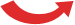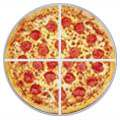# Using Rational Numbers

How to add, subtract, multiply and divide rational numbers

A rational number is a number that can be written as a simple fraction (i.e. as a ratio).

### Examples:

Number As a Fraction
5 5/1
1.75 7/4
.001 1/1000
0.111... 1/9

## In general ...

So a rational number looks like this:

pq

But q cannot be zero, as that is dividing by zero.

## How to Add, Subtract, Multiply and Divide

When the rational number is something simple like 3, or 0.001, then just use mental arithmetic, or a calculator!

But what about when it is in pq form?

 ½ Well, a rational number is a fraction, so we can use:

Here we will see those operations in a more general Algebra style.

You might also like to read Fractions in Algebra.

## Multiplication

To multiply two rational numbers multiply the tops and bottoms separately, like this:

Here is an example:

## Division

To divide two rational numbers, first flip the second number over (make it a reciprocal) and then do a multiply like above:

Here is an example:

We will cover Addition and Subtraction in one go, as they are the same method.

Before we add or subtract, the rational numbers should have the same bottom number (called a Common Denominator).

The easiest way to do this is to

Multiply both parts of each number by the bottom part of the other

Like this (note that the dot · means multiply):

Here is an example of addition:

And an example of subtraction (the middle step is skipped to make it quicker):

## Simplest Form

Sometimes we have a rational number like this:

1015

But that is not as simple as it can be!

We can divide both top and bottom by 5 to get:

 ÷ 51015   =   23÷ 5

Now it is in "simplest form", which is how most people want it!

## Be Careful With "Mixed Fractions"

We may be tempted to write an Improper Fraction (a fraction that is "top-heavy", i.e. where the top number is bigger then the bottom number) as a Mixed Fraction:

For example 7/4 = 1 3/4, shown here:Improper FractionMixed Fraction 7/4 1 3/4=But for mathematics the "Improper" form (such as 7/4) is actually better.

Because Mixed fractions (such as 1 3/4) can be confusing when we write them down in a formula, as it can look like a multiplication:

Mixed Fraction:   Improper Fraction: What is: 1 + 214 ? Is it: 1 + 2 + 14 = 314 ? Or is it: 1 + 2 × 14 = 112 ? What is: 1 + 94 ? It is: 44 + 94 = 134So try to use the Improper Fraction when doing mathematics.